7. Kinematics-Dynamics of Non-uniform Circular Motion-Rest

L. G. Kreidik (translation from Russian T. S. Kortneva and G. P. Shpenkov)

In a general case of non-uniform revolution of the system of material points, potential-kinetic projections of motion of the points along the axes x and y has the form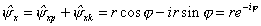, (2.166)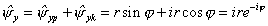, (2.166Ó)

where r is the distance to the axis of revolution, cp is the angular displacement.

The potential-kinetic velocity of motion of the points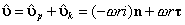(2.167)

has a structure of uniform motion but acceleration here acquires an additional term, reflecting the non-uniform aspect of the motion: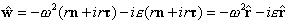, (2.168)

where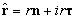is a potential-kinetic radius.

The first term in the right-hand side is qualitative acceleration, acceleration of self-motion, a measure of uniform motion. The second term is quantitative acceleration, acceleration of non-self-motion, a measure of non-uniform motion. Thus, non-uniform circular motion is contradictory, being uniform-non-uniform. This statement is evidently valid for any non-uniform motion.

The terms in the expression for acceleration (2.168) will be rearranged as follows: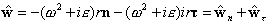, (2.169)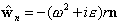(2.169Ó)

is normal potential-kinetic acceleration;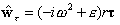(2.169b)

is tangential potential-kinetic acceleration.

In expression (2.169a), the first term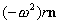is the centripetal potential acceleration, the second one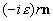is the normal kinetic acceleration (Fig. 2.8).

In tangential acceleration (2.169b), the first term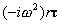is the tangential kinetic acceleration, the second term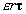is the tangential potential acceleration.

Now we will introduce the acceleration as the qualitatively-quantitative sum: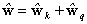, (2.170)

where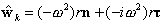. (2.170Ó)

is a qualitative component of the acceleration;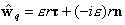(2.170b)

is a quantitative component of its.

The qualitative acceleration (2.170a) is the potential-kinetic centripetal acceleration, whereas the quantitative acceleration (2.170b) is the potential-kinetic tangential acceleration.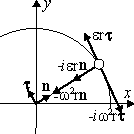Fig. 2.8. Accelerations in non-uniform circular motion-rest or a vector graph of accelerations.

We will consider as well the potential-kinetic structure of the acceleration: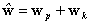, (2.171)

where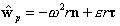(2.171a)

is the potential acceleration;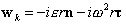(2.171b)

is the kinetic acceleration.

Specific acceleration has a similar structure. In particular, a normal-tangential or transverse specific acceleration has the form: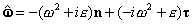. (2.172)

Consequently, the longitudinal-transverse kinema of circular rest-motion is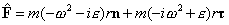, (2.173)

where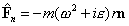(2.173a)

is the normal or longitudinal potential-kinetic kinema;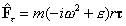(2.173b)

is the tangential or transverse potential-kinetic kinema (Fig. 2.9).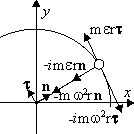Fig. 2.9. A vector graph of kinemas in non-uniform

The kinema defines the longitudinal-transverse moment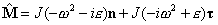, (2.174)

where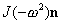and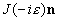are centripetal moments of rest-motion;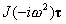and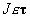are tangential moments of rest-notion.

The sum of moments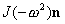+defines uniform rotation and+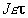non-uniform rotation.

The axial moment in non-uniform rotation is defined by the equality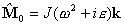. (2.175)

In classical physics, the tangential potential momentis used only in the form of the axial moment with the norm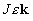.

Kinematic non-uniform current is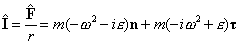(2.176)

or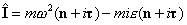. (2.176a)

The laws of motion in circular motion are similar to the respective laws in rectilinear motion. In particular, the law of conservation of absolute-relative energy in the case of elastic-inelastic impact in rotational motion has the form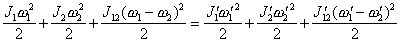. (2.177)

Theoretical Dialectical Journal: Physics-Mathematics-Logic-Philosophy, N.2, site http://www.tedial.narod.ru/